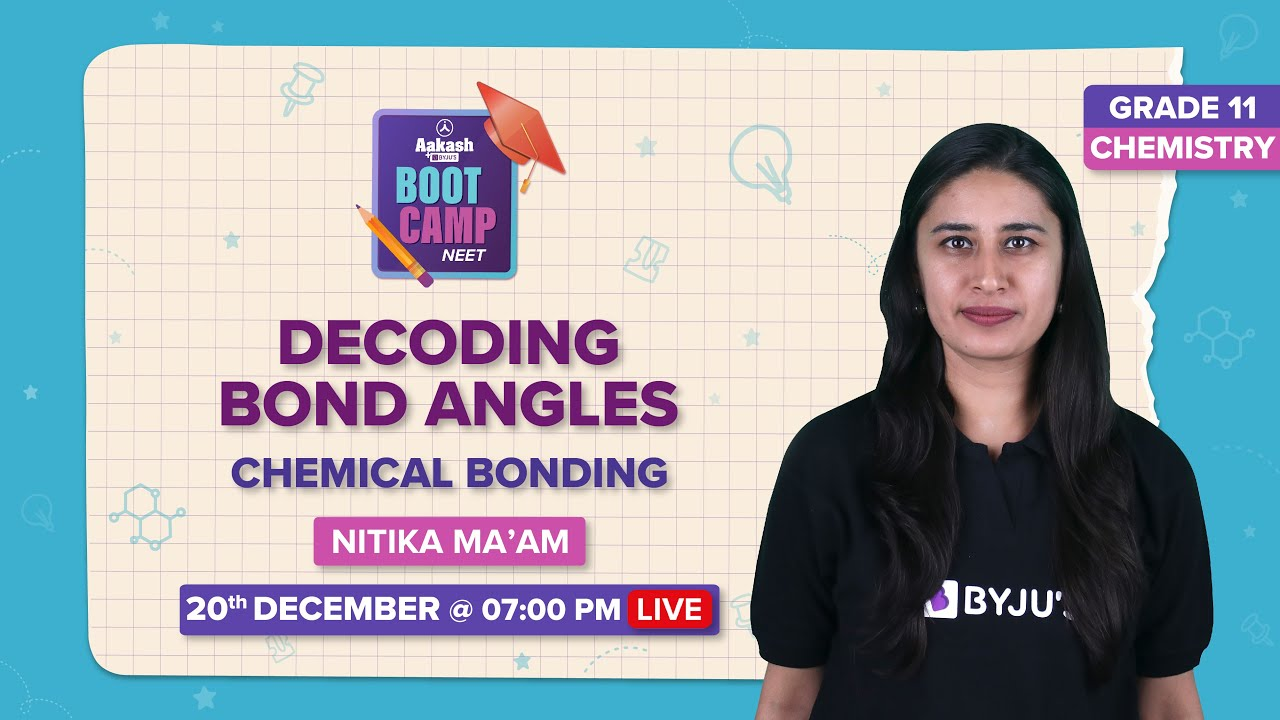# Flashcards for NEET Chemistry - Some Basic Concepts of Chemistry

Flashcards for NEET Chemistry are designed to boost your NEET preparation. Find below flashcards for the chapter “Some Basic Concepts of Chemistry”. These flashcards are prepared as per the NEET syllabus. These are helpful for aspirants of NEET and other exams during last-minute revision. It covers all the important points that are frequently asked in the exam. Check BYJU’S for the full set of Flashcards and Study material for NEET Chemistry.

## Download PDF of NEET Chemistry Flashcards for Some Basic Concepts of Chemistry

 Name of the NEET Sub-section Topic Flashcards Helpful for Chemistry Some Basic Concepts of Chemistry NEET exams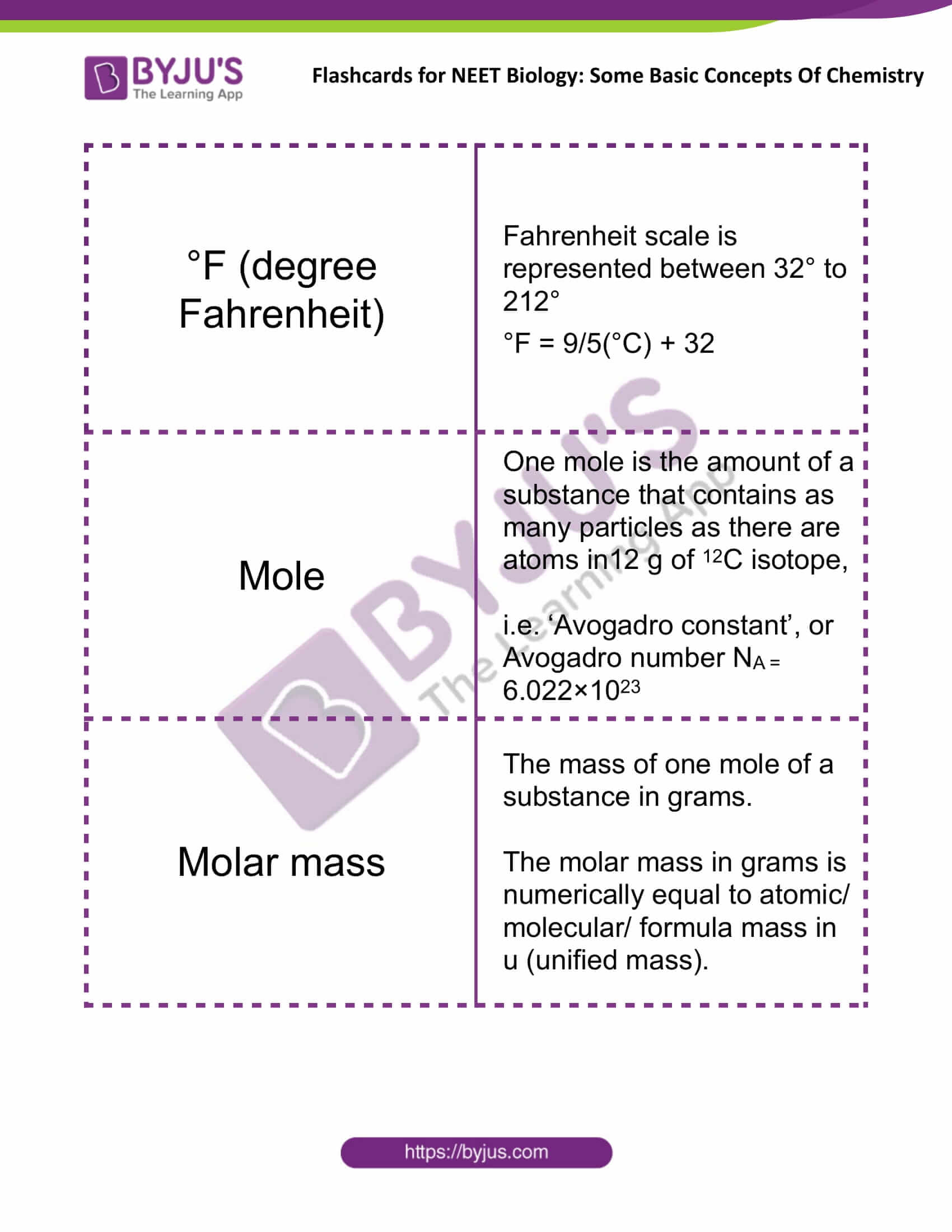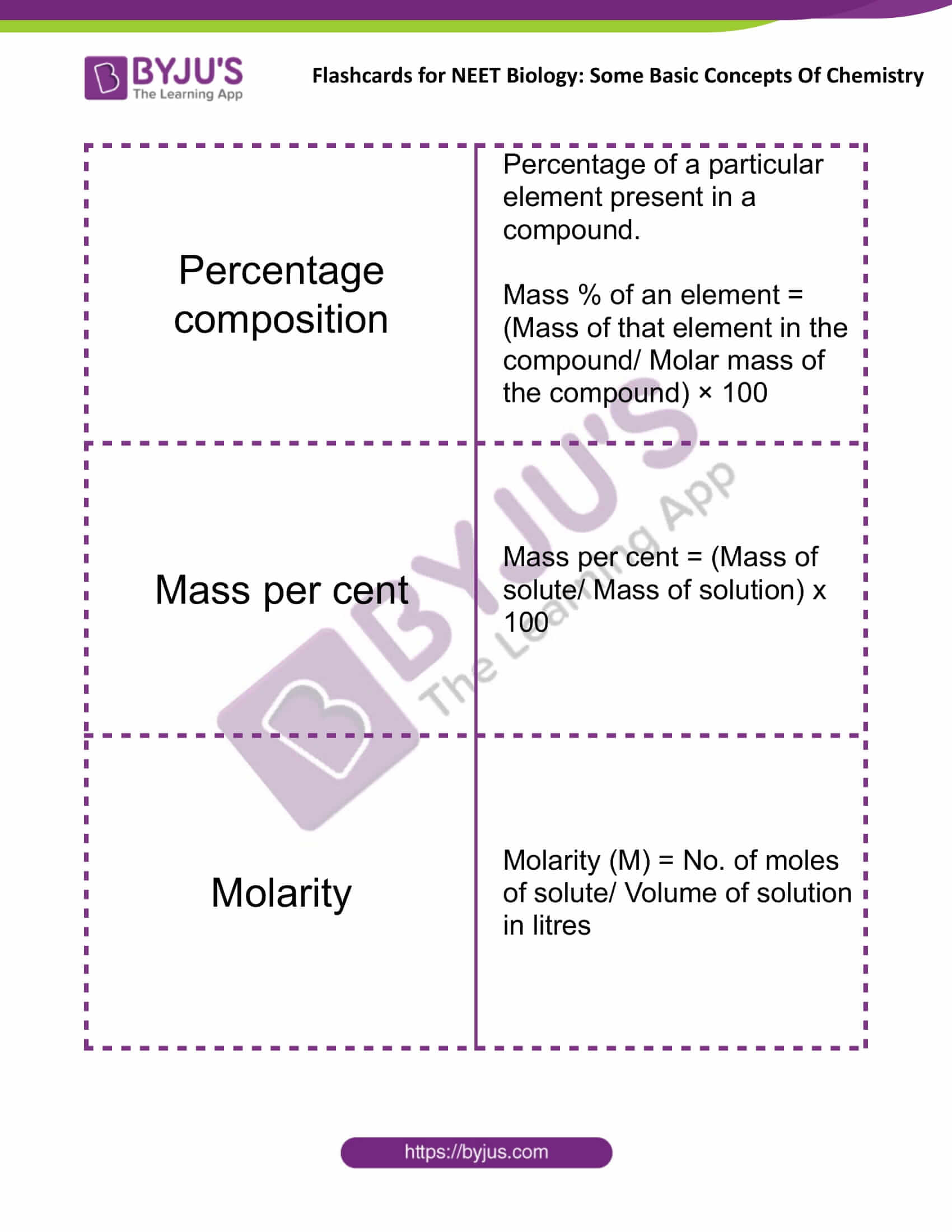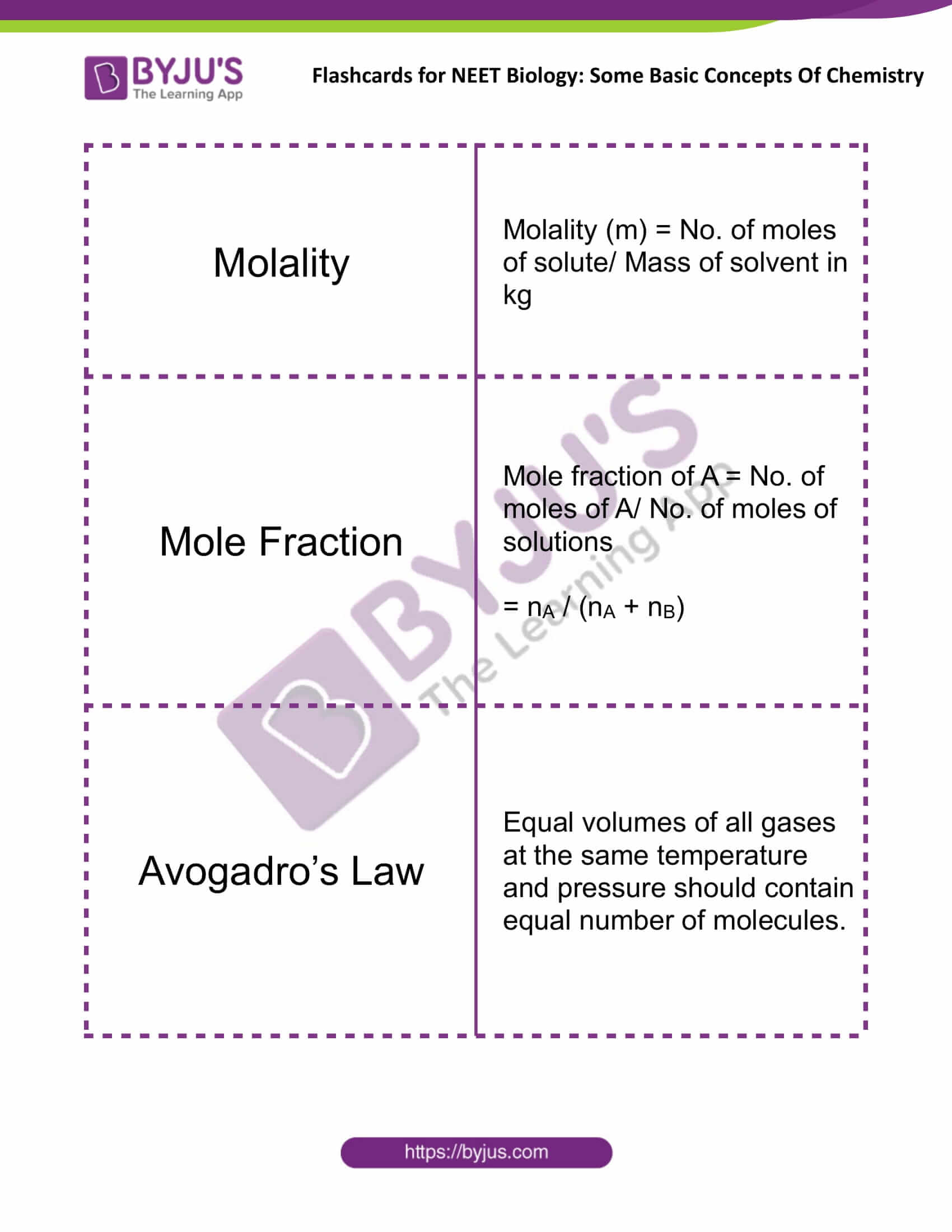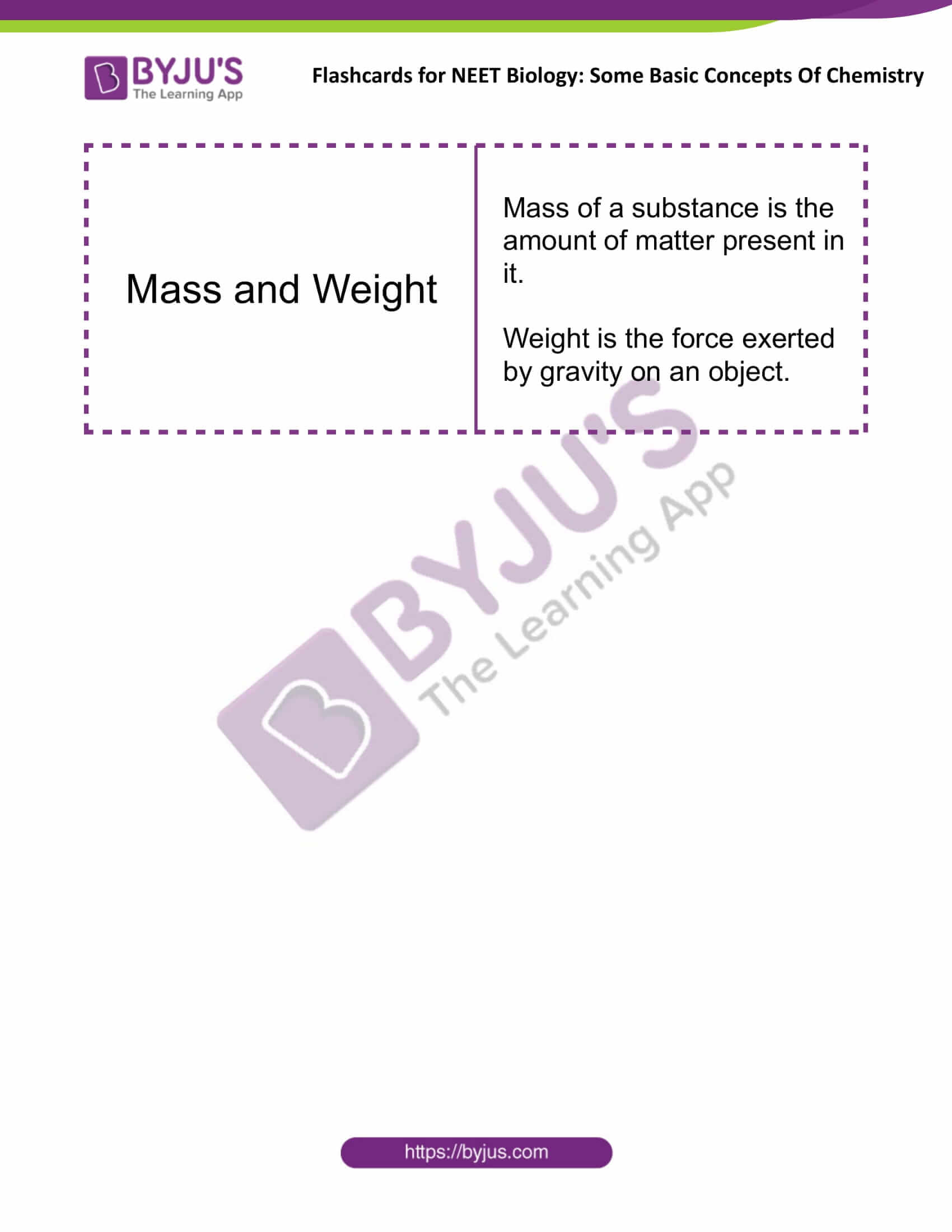Some Basic Concepts of Chemistry °F (degree Fahrenheit) Fahrenheit scale is represented between 32° to 212° °F = 9/5(°C) + 32 Mole One mole is the amount of a substance that contains as many particles as there are atoms in12 g of 12C isotope, i.e. ‘Avogadro constant’, or Avogadro number NA = 6.022×1023 Molar Mass The mass of one mole of a substance in grams. The molar mass in grams is numerically equal to atomic/molecular/formula mass in u (unified mass). Percentage Composition Percentage of a particular element present in a compound. Mass % of an element = (Mass of that element in the compound/Molar mass of the compound) × 100 Mass Percent Mass percent = (Mass of solute/Mass of solution) x 100 Molarity Molarity (M) = No. of moles of solute/ Volume of solution in litres Molality Molality (m) = No. of moles of solute/ Mass of solvent in kg Mole Fraction Mole fraction of A = No. of moles of A/ No. of moles of solutions = nA / (nA + nB) Avogadro’s Law Equal volumes of all gases at the same temperature and pressure should contain an equal number of molecules. Mass and Weight The mass of a substance is the amount of matter present in it. Weight is the force exerted by gravity on an object.

Get access to the full set of flashcards for NEET Chemistry, only at BYJU’S.

### Recommended Video:

NEET 2022: Chemical Bonding & Bond Angles | Class 11 Chemistry | Aakash BYJU’S NEET Bootcamp